# Linear Equations Exercise 3.2

In this page we have NCERT Solutions for Class 10 Maths Linear Equations for Exercise 3.2 on pages 49 and 50. Hope you like them and do not forget to like , social_share and comment at the end of the page.
The graph of a pair of linear equations in two variables is represented by two lines.
1. If the lines intersect at a point, then that point gives the unique solution of the two equations. Then the pair of equations is consistent.
2. If the lines coincide, then there are infinitely many solutions - each point on the line being a solution. Then the pair of equations is dependent (consistent).
3. If the lines are parallel, then the pair of equations has no solution. In this case, the pair of equations is inconsistent

For pair of linear equation
$a_1x+b_1y+c_1= 0$
$a_2x+b_2y+c_2= 0$
if $\frac {a_1}{a_2} \ne \frac {b_1}{b_2}$ then the pair of equations is consistent
if $\frac {a_1}{a_2} = \frac {b_1}{b_2}= \frac {c_1}{c_2}$,then the pair of equations is consistent and dependent
if $\frac {a_1}{a_2} = \frac {b_1}{b_2} \ne \frac {c_1}{c_2}$,then the pair of equations is inconsistent

## NCERT Solutions for Class 10 Maths Chapter 3 Exercise 3.2

Question 1
Form the pair of linear equations in the following problems, and find their solutions graphically.

(i) 10 students of Class X took part in a Mathematics quiz. If the number of girls is 4 more than the number of boys, find the number of boys and girls who took part in the quiz.
(ii) 5 pencils and 7 pens together cost Rs 50, whereas 7 pencils and 5 pens together cost Rs 46. Find the cost of one pencil and that of one pen.

Solution
(i) Let number of boys =x
Number of girls =y
Given that total number of student is 10 so that
$x + y= 10$
or
$y= 10-x$
Putting x= 10, 0 we get

 x 10 0 y 0 10

Given that If the number of girls is 4 more than the number of boys
So that
$y=x+ 4$
Putting x= -4, 0 and we get

 x -4 0 y 0 4

Graphical representation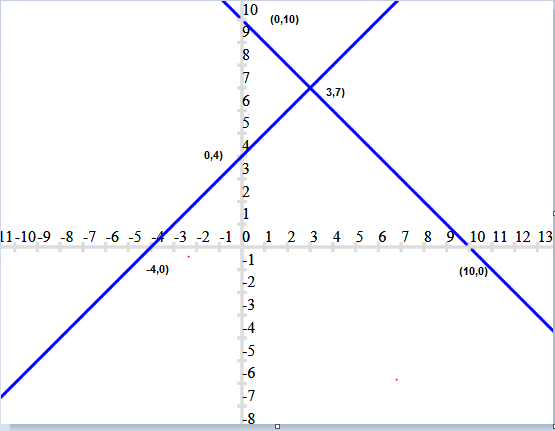Therefore, number of boys = 3 and number of girls = 7.
(ii) Let cost of pencil = Rs x
Cost of pens = Rs y
5 pencils and 7 pens together cost Rs 50,
So we get
$5x+ 7y= 50$
or
$y= \frac {50 - 5 x} {7}$
Here putting x=0, will give fractional value of y which will be difficult to drawn in graph, so we take values such that, we get integers values
Putting value of x= 3 and -4 we get

 x 3 -4 y 5 10

Given that 7 pencils and 5 pens together cost Rs 46
$7x+ 5y= 46$
$y= \frac {46 - 7x}{5}$

Putting x= -2, 3 we get

 x -2 3 y 12 5

Graphical representation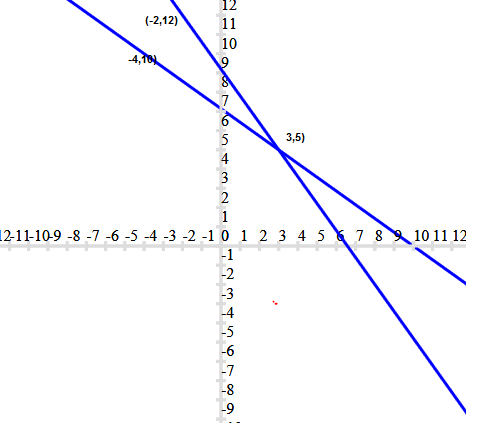Therefore, cost of one pencil = Rs 3 and cost of one pen= Rs 5.

Question 2
On comparing the ratios a1/a2,b1/b2 and c1/c2, find out whether the lines representing the following pairs of linear equations intersect at a point, are parallel or coincident.
(i) $5x - 4y+ 8 = 0$
$7x+ 6y- 9 = 0$
(ii) $9x+ 3y+ 12 = 0$
$18x+ 6y+ 24 = 0$

(iii) $6x- 3y+ 10 = 0$
$2x -y+ 9 = 0$

(i) Comparing these equation with
$a_1x+b_1y+c_1= 0$
$a_2x+b_2y+c_2= 0$
We get
a1= 5,b1= -4, and c1= 8
a2=7,b2= 6 and c2= -9
a1/a2= 5/7,
b1/b2= -4/6 and
c1/c2= 8/-9
Hence,a1/a2≠ b1/b2
Therefore, both are intersecting lines at one point.
(ii)Comparing these equations with
$a_1x+b_1y+c_1= 0$
$a_2x+b_2y+c_2= 0$
We get
a1= 9,b1= 3, and c1= 12
a2= 18,b2= 6 and c2= 24
a1/a2= 9/18 = 1/2
b1/b2= 3/6 = 1/2 and
c1/c2= 12/24 = 1/2
Hence,a1/a2=b1/b2=c1/c2

Therefore, both lines are coincident
(iii) Comparing these equations with
$a_1x+b_1y+c_1= 0$
$a_2x+b_2y+c_2= 0$
We get
a1= 6,b1= -3, and c1= 10
a2= 2,b2= -1 and c2= 9
a1/a2= 6/2 = 3/1
b1/b2= -3/-1 = 3/1 and
c1/c2= 12/24 = 1/2
Hence,a1/a2=b1/b2≠c1/c2

Therefore, both lines are parallel

Question 3
On comparing the ratios a1/a2,b1/b2 and c1/c2, find out whether the following pair of linear equations are consistent, or inconsistent.
(i) $3x+ 2y= 5$; $2x- 3y= 7$
(ii) $2x - 3y= 8$; $4x - 6y= 9$
(iii) $\frac {3}{2} x+ \frac {5}{3} y= 7$; $9x- 10y= 14$
(iv) $5x -3y= 11$; $-10x+ 6y= -22$
(v) $\frac {4}{3}x+ 2y=8$; $2x+ 3y= 12$
(i) $3x+ 2y= 5$; $2x- 3y= 7$
Comparing these equations with
$a_1x+b_1y+c_1= 0$
$a_2x+b_2y+c_2= 0$
We get

a1/a2= 3/2
b1/b2= -2/3and
c1/c2= 5/7
Hence,a1/a2≠ b1/b2
These linear equations are intersecting each other at one point and thus have only one possible solution. Hence, the pair of linear equations is consistent.
(ii) $2x - 3y= 8$; $4x - 6y= 9$
Comparing these equations with
$a_1x+b_1y+c_1= 0$
$a_2x+b_2y+c_2= 0$
We get

a1/a2= 2/4 = 1/2
b1/b2= -3/-6= 1/2 and
c1/c2= 8/9
Hence,a1/a2=b1/b2≠c1/c2

Therefore, these linear equations are parallel to each other and thus have no possible solution. Hence, the pair of linear equations is inconsistent.

(iii)$\frac {3}{2} x+ \frac {5}{3} y= 7$; $9x- 10y= 14$
Comparing these equations with
$a_1x+b_1y+c_1= 0$
$a_2x+b_2y+c_2= 0$
We get

a1/a2= 3/2/9= 1/6
b1/b2= 5/3/-10 = -1/6 and
c1/c2= 7/14 = 1/2
Hence,a1/a2≠b1/b2

Therefore, these linear equations are intersecting each other at one point and thus have only one possible solution. Hence, the pair of linear equations is consistent.
(iv) $5x -3y= 11$; $-10x+ 6y= -22$
Comparing these equations with
$a_1x+b_1y+c_1= 0$
$a_2x+b_2y+c_2= 0$
We get

a1/a2= 5/-10= -1/2
b1/b2= -3/6= -1/2 and
c1/c2= 11/-22 = -1/2
Hence,a1/a2=b1/b2=c1/c2

Therefore, these linear equations are coincident pair of lines and thus have infinite number of possible solutions. Hence, the pair of linear equations is consistent.
(v) $\frac {4}{3}x+ 2y=8$; $2x+ 3y= 12$
Comparing these equations with
$a_1x+b_1y+c_1= 0$
$a_2x+b_2y+c_2= 0$
We get

a1/a2= 4/3/2= 2/3
b1/b2=2/3 and
c1/c2= 8/12 = 2/3
Hence,a1/a2=b1/b2=c1/c2

Therefore, these linear equations are coincident pair of lines and thus have infinite number of possible solutions. Hence, the pair of linear equations is consistent.
Question 4
Which of the following pairs of linear equations are consistent/inconsistent? If consistent, obtain the solution graphically:
(i) $x+y= 5$, $2x+ 2y= 10$
(ii) $x -y= 8$, $3x- 3y= 16$
(iii) $2x+y- 6 = 0$, $4x- 2y- 4 = 0$
(iv) $2x -2y- 2 = 0$, $4x- 4y- 5 = 0$
(i)$x+y= 5$, $2x+ 2y= 10$
Comparing these equations with
$a_1x+b_1y+c_1= 0$
$a_2x+b_2y+c_2= 0$
We get

a1/a2= 1/2
b1/b2=1/2 and
c1/c2= 5/10 = 1/2
Hence,a1/a2=b1/b2=c1/c2
Therefore, these linear equations are coincident pair of lines and thus have infinite number of possible solutions. Hence, the pair of linear equations is consistent.

x+y= 5
or y= 5 -x
 x 0 5 y 5 0

And, 2x+ 2y= 10
or y= 5-x
This is same as Ist equation only
 x 0 5 y 5 0

Graphical representation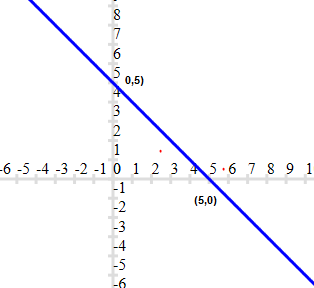(ii)x–y= 8, 3x– 3y= 16
Comparing these equations with
$a_1x+b_1y+c_1= 0$
$a_2x+b_2y+c_2= 0$
We get

a1/a2= 1/3
b1/b2= -1/-3 = 1/3 and
c1/c2= 8/16 = 1/2
Hence,a1/a2=b1/b2≠c1/c2
Therefore, these linear equations are parallel to each other and thus have no possible solution. Hence, the pair of linear equations is inconsistent.
(iii) 2x+y– 6 = 0, 4x– 2y– 4 = 0
Comparing these equations with
$a_1x+b_1y+c_1= 0$
$a_2x+b_2y+c_2= 0$
We get

a1/a2= 2/4 = 1/2
b1/b2= -1/2 and
c1/c2= -6/-4= 3/2
Hence,a1/a2≠ b1/b2

Therefore, these linear equations are intersecting each other at one point and thus have only one possible solution. Hence, the pair of linear equations is consistent.
2x+y- 6 = 0
y= 6 - 2x

 x 0 3 y 6 0

And, 4x- 2y-4 = 0
y= 2x- 2
 x 0 1 y -2 0

Graphical representation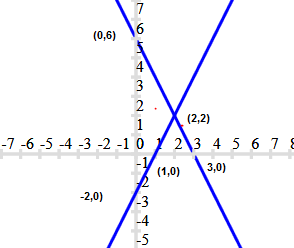From the figure, it can be observed that these lines are intersecting each other at the only one point i.e., (2,2) which is the solution for the given pair of equations.
(iv) 2x– 2y– 2 = 0, 4x– 4y– 5 = 0
Comparing these equations with
$a_1x+b_1y+c_1= 0$
$a_2x+b_2y+c_2= 0$
We get

a1/a2= 2/4 = 1/2
b1/b2= -2/-4 = 1/2 and
c1/c2= 2/5
Hence,a1/a2=b1/b2≠c1/c2

Therefore, these linear equations are parallel to each other and thus, have no possible solution. Hence, the pair of linear equations is inconsistent.
Question 5
Half the perimeter of a rectangular garden, whose length is 4 m more than its width, is 36 m. Find the dimensions of the garden.
Let length of rectangle =x m
Width of the rectangle =y m
According to the question,
y-x= 4 ...(i)
y+x= 36 ...(ii)
Now
y=x+ 4
 x 0 -4 y 4 0

y= 36 -x
 x 0 36 y 36 0

Graphical representation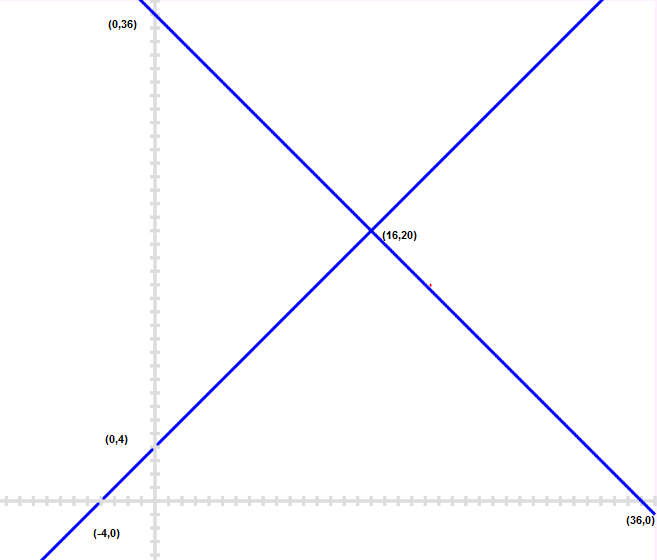From the figure, it can be observed that these lines are intersecting each other at only point i.e., (16, 20). Therefore, the length and width of the given garden is 20 m and 16 m respectively.
Question 6
Given the linear equation $2x+ 3y- 8 = 0$, write another linear equation in two variables such that the geometrical representation of the pair so formed is:
(i) intersecting lines
(ii) parallel lines
(iii) coincident lines

(i) Intersecting lines:
For this condition,
a1/a2≠ b1/b2
The second line such that it is intersecting the given line is
$2x+ 5y- 7 = 0$ as
a1/a2= 2/2= 1
b1/b2= 3/5 and
a1/a2≠ b1/b2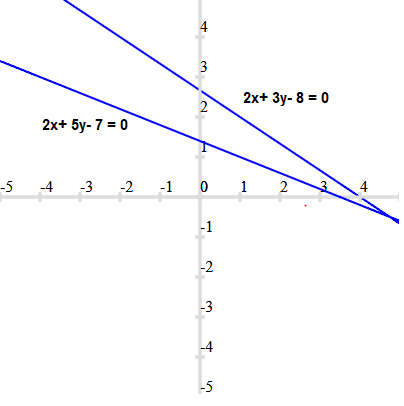(ii) Parallel lines
For this condition,
a1/a2=b1/b2≠c1/c2
Hence, the second line can be
$6x+ 9y- 8 = 0$ as
a1/a2= 2/6= 1/3
b1/b2= 3/9 = 1/3 and
c1/c2= -8/-8 = 1
and a1/a2=b1/b2≠c1/c2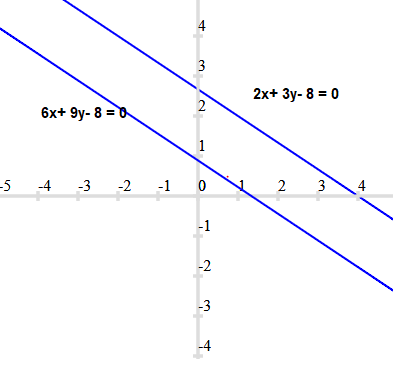(iii) Coincident lines
For coincident lines,
a1/a2=b1/b2=c1/c2
Hence, the second line can be
$8x+ 12y = 32$ as
a1/a2= 2/8= 1/4
b1/b2= 3/12= 1/4 and
c1/c2= -8/-24 = 1/4
and a1/a2=b1/b2=c1/c2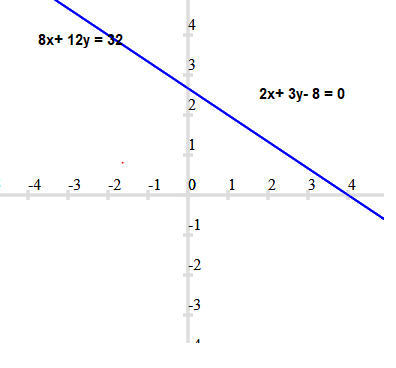Question 7
Draw the graphs of the equations $x- y + 1 = 0$ and $3x+ 2y- 12 = 0$. Determine the coordinates of the vertices of the triangle formed by these lines and the x-axis, and shade the triangular region.

$x-y+ 1 = 0$
$y=x+ 1$
 x 0 -1 y 1 0

$3x+ 2y- 12 = 0$
$y= 6 - \frac {3}{2} x$
 x 4 0 y 0 6

Graphical representation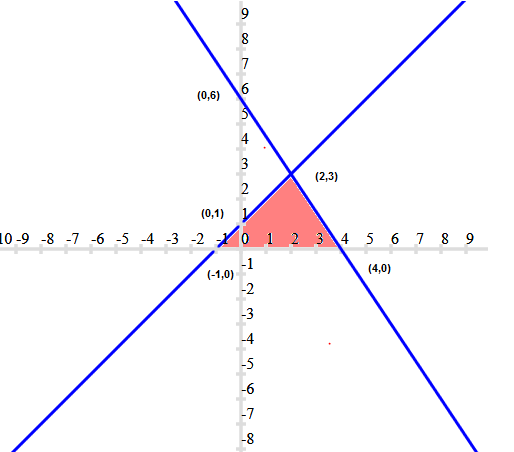From the figure, it can be observed that these lines are intersecting each other at point (2, 3) and x-axis at (- 1, 0) and (4, 0). Therefore, the vertices of the triangle are (2, 3), (- 1, 0), and (4, 0).

## Summary

1. NCERT Solutions for Class 10 Maths Chapter 3 Linear Equations Exercise 3.2 has been prepared by Expert with utmost care. If you find any mistake.Please do provide feedback on mail.
2. This chapter 3 has total 7 Exercise 3.1 ,3.2,3.3,3.4,3.5,3.6 and 3.7. This is the Second exercise in the chapter.You can explore previous exercise of this chapter by clicking the link belowGo back to Class 10 Main Page using below links

### Practice Question

Question 1 What is $1 - \sqrt {3}$ ?
A) Non terminating repeating
B) Non terminating non repeating
C) Terminating
D) None of the above
Question 2 The volume of the largest right circular cone that can be cut out from a cube of edge 4.2 cm is?
A) 19.4 cm3
B) 12 cm3
C) 78.6 cm3
D) 58.2 cm3
Question 3 The sum of the first three terms of an AP is 33. If the product of the first and the third term exceeds the second term by 29, the AP is ?
A) 2 ,21,11
B) 1,10,19
C) -1 ,8,17
D) 2 ,11,20